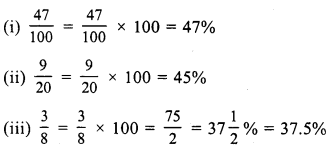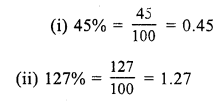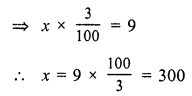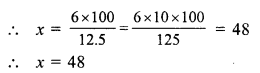# RS Aggarwal Class 7 Solutions Chapter 10 Percentage Ex 10A

In this chapter, we provide RS Aggarwal Solutions for Class 7 Chapter 10 Percentage Ex 10A for English medium students, Which will very helpful for every student in their exams. Students can download the latest RS Aggarwal Solutions for Class 7 Chapter 10 Percentage Ex 10A Maths pdf, free RS Aggarwal Solutions Class 7 Chapter 10 Percentage Ex 10A Maths book pdf download. Now you will get step by step solution to each question.

### RS Aggarwal Solutions for Class 7 Chapter 10 Percentage Ex 10A Download PDF

Question 1.
Solution:Question 2.
Solution:Question 3.
Solution:Question 4.
Solution:Question 5.
Solution:Question 6.
Solution:Question 7.
Solution:Question 8.
Solution:Question 9.
Solution:
Let x is the required numberQuestion 10.
Solution:
Let x be the required numberQuestion 11.
Solution:
10 % of Rs. 90 = 90 x 10100 = Rs. 9
Required amount = Rs. 90 + Rs. 9 = Rs. 99

Question 12.
Solution:
20 % of Rs. 60 = 60×20100 = 12
Required amount = Rs. 60 – 12 = Rs. 48

Question 13.
Solution:
3 % of x = 9Question 14.
Solution:
12.5 % of x = 6
⇒ x x 12.5100 = 6Question 15.
Solution:
Let x % of 84 = 14Question 16.
Solution:All Chapter RS Aggarwal Solutions For Class 7 Maths

—————————————————————————–

All Subject NCERT Exemplar Problems Solutions For Class 7

All Subject NCERT Solutions For Class 7

*************************************************

I think you got complete solutions for this chapter. If You have any queries regarding this chapter, please comment on the below section our subject teacher will answer you. We tried our best to give complete solutions so you got good marks in your exam.

If these solutions have helped you, you can also share rsaggarwalsolutions.in to your friends.